# GRE Subject Test: Chemistry : Oxidation-Reduction Analysis

## Example Questions

### Example Question #1 : Oxidation Reduction Analysis

Which molecule would be considered a Lewis acid?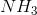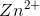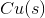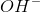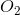Explanation:

A lewis acid is an electron pair acceptor.would be considered a lewis acid based on the definition. Becauseis electron-deficient based on its oxidation number, it is able to accept an electron pair.

### Example Question #12 : Analyzing Reactions

Which of the following would be considered a Lewis base?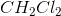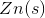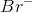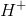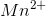Explanation:

A lewis base is an electron pair donor.would be considered a lewis base based on the definition. Becauseis electron rich based on its oxidation number, it is able to donate an electron pair to an electron deficient ion.

### Example Question #13 : Analyzing Reactions

Which of the following types of reactions best describes the following reaction: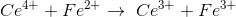Combustion

Redox reaction

Catalytic

Combination

Double replacement

Redox reaction

Explanation:

A redox reaction is also known as an oxidation/reduction reaction. This type of reaction involves the transfer of electrons from on reactant to another. In this reaction, an electron is transferred from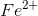to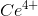forming the products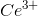and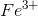. The substance that gains an electron is referred to as being reduced. The substance that loses an electron is referred to as being oxidized.

### Example Question #1 : Oxidation Reduction Analysis

What is the oxidation state of manganese in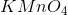?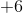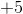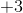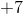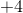Explanation:

In this compound oxygen has an oxidation number of. There are 4 oxygens, therefore the total oxidation number for the oxygens inis: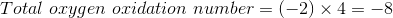. Potassium,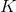, is a group 1 metal and has an oxidation number of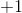. The sum of all oxidation number in a neutral compound such asis zero. We will give the oxidation number of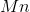equal to: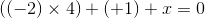Simplifying the above equation: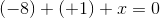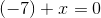Rearranging to solve for: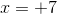### Example Question #15 : Analyzing Reactions

What is the oxidation state of manganese in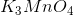?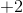Explanation:

In this compound oxygen has an oxidation number of. There are 4 oxygens, therefore the total oxidation number for the oxygens inis: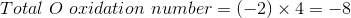. Potassium,, is a group 1 metal and has an oxidation number of. There are 3 potassium ions present in, therefore the total oxidation number for the potassium ions is: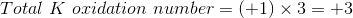.The sum of all oxidation number in a neutral compound such asis zero. We will give the oxidation number ofequal to: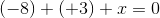Simplifying the above equation: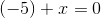Rearranging to solve for: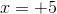### All GRE Subject Test: Chemistry Resources